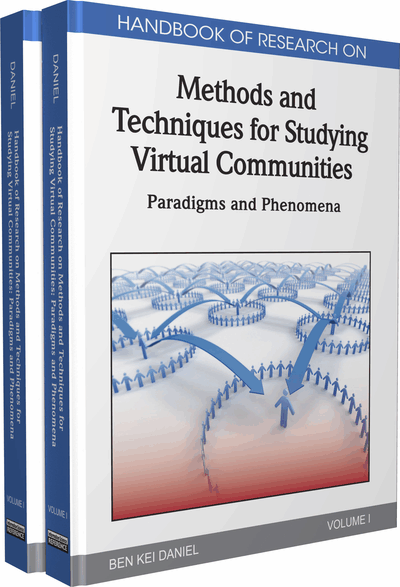# Mathematical Retrieval Techniques for Online Mathematics Learning

Le Van Tien (Hochiminh City University of Technology, Vietnam), Quan Thanh Tho (Hochiminh City University of Technology, Vietnam) and Hui Siu Cheung (Nanyang Technological University, Singapore)
DOI: 10.4018/978-1-60960-040-2.ch024
Available
\$37.50
No Current Special Offers

## Abstract

In recent years, the number of computer-aided educational softwares in mathematics field has been increasing. Currently, there are some research prototypes and systems assisting finding mathematical problems. However, when finding appropriate mathematical expressions, most of these systems only support mechanisms to search expression in a strict exact manner, or search some similar problems based on wildcard, not on the similarity of expression structures and semantic meanings. Such mechanisms restrict users significantly from achieving meaningful and accurate search results of mathematical expressions. In this chapter, we introduce a mathematical retrieval system that helps mathematics learners self-study effectively. The most important module in our system is the math-retrieving system module, which received the analyzed problems submitted from users, retrieves solutions from similar stored problems and ranks the retrieved problems to users. To fulfill these requirements, we have researched and proposed some advanced mathematical retrieval and mathematical ranking techniques. Experiments have shown that our proposed techniques highly suitable for mathematical retrieval as they outperformed the techniques used in typical document retrieval system
Chapter Preview
Top

## Introduction

In recent years, Information Retrieval has emerged as a significant field in terms of both research and development for many prototypes and applications intended to process information on the Internet. The names such as Google (Google), Yahoo! Search (Yahoo), Baidu (Dawn, 2007) and Bing (Schofield, 2009) have become familiar to Internet users nowadays. These search engines have attracted the increased numbers of Internet users because of their powerful capabilities of finding information quickly over the huge resources available on the World Wide Web. However, the current generation of search engines has shown some prominent shortcomings in searching semantic information. For example, with the query “Where is the Sun Flower?”, it is not easy to infer the real semantics associated with the term “Sun Flower”, which can be a kind of flower or a company name. Clearly, this question cannot be answered precisely if we are only merely based on the word lexicon. Thus, the need of a search engine which can search semantic content effectively becomes highly desirable, which introduces the recently emerging semantic search engine Wolfram Alpha (Johnson, 2009).

This research investigates mining and retrieving semantic information on another type of data, rather than the textual one. In our system, we have attempted to build a module that can retrieve the mathematical content precisely. One important advantage of mathematical data is that it conveys higher semantic level than that of textual data. For example, when encountering the term log appearing in a mathematical expression, we can be certain about the semantic meaning associated with this term, which is the arithmetic logarithm function.

Currently, there are some research prototypes and systems assisting finding mathematical problems such as MathWebSearch (Kohlhase & Sucan, 2006) and MathDex (Miner & Munavalli, 2007). However, when finding appropriate mathematical expressions, most of these systems only support mechanisms to search expression in a strict exact manner, or search some similar problems based on wildcard, not on the similarity of expression structures and semantic meanings. Such mechanisms restrict users significantly from achieving meaningful and accurate search results of mathematical expressions. Therefore, a mathematics search engine based on similarity of mathematical expressions has important value in helping mathematics learners approach desirable solutions quickly.

In this chapter we also discuss applying mathematics search engine in the education domain, rather than merely technical aspects. Thus, we propose a mathematical retrieval system that helps mathematics learners self-study effectively. The proposed system consists of the following major modules. First, a math-browser module has been developed to help learners browse classes of mathematics problems in a friendly and organized manner. Next, a testing module has also been built to enable learners to take a trial test and get the results online. Lastly, a math-retrieving module has been constructed to assist users on solving exercises based on some information retrieval techniques. This module can help users find similar problems when trying to solve certain specific problems. In addition, the system can get input of mathematical expressions from users in a friendly hand-writing manner. During the development of such a system, we have researched and employed the following advanced mathematics retrieval techniques:

• Mathematical retrieval: we have proposed a technique to process and retrieve mathematical data, adapted from the typical vector space model and tfidf weights that are widely used for document retrieval (Baeza-Yates & Ribeiro-Neto, 1999).

• Mathematical ranking: While the adapted tfidf technique is useful for retrieving mathematical problems, it is not efficient to rank the retrieved problem due the specific meaning implied by mathematical symbols and formulas. Thus, we develop a graph-based matching approach for the ranking problem. Our approach suggests a mixture between the Hungarian algorithm (Kuhn, 1956) with a self-developed tree-based matching algorithm to deal with variety of mathematical problems ranged in different levels of complexity.

## Key Terms in this Chapter

Mathematical Retrieval: To retrieve mathematical contents based on a submitted query.

Retrieval Ranking: To rank the information retrieved based on the relevance/ similarity with the submitted query.

Tree-matching ranking: To rank the retrieved mathematics expression based on the similarities between their corresponding tree-like representatives with that of the original query.

Information Retrieval: To retrieve information from a dataset based on a submitted query.

Vector Space Model: To model documents in a dataset as numerical vectors for the sake of retrieval.

Matching Problem: To match two graphs in order to infer the similarity between them.

Tf•idf weightWeight: The weight indicating the importance/significance of a term in a document.

## Complete Chapter List

Search this Book:
Reset